# C180 – COSTALD-Tait Density Calculation

## Description

This COSTALD calculation comprises four distinct density calculation options.

The “standard” COSTALD equation is used to calculate the saturated liquid density of light hydrocarbon mixtures (LPGs) from composition.

The “enhanced” COSTALD equation, which differs only slightly from the “standard” COSTALD equation, is used to calculate the saturated liquid density of LNG mixtures (i.e. predominantly CH4).

The Tait extension to the COSTALD equation (known as COSTALD-Tait) calculates the compressed liquid density of light hydrocarbon mixtures (i.e. density at pressures above the saturation pressure). The Tait extension applies to both the “standard” and “enhanced” COSTALD equations giving four options in total.

IP Petroleum Manual. Part XII – Static and Dynamic Measurement of Light Hydrocarbon Liquids. Section 1 – Calculation Procedures. 

ISO 6578:1991 (BS 7577:1992) – Annex G. 

KELTON calculation reference C180

FLOCALC calculation reference F059

KIMS calculation references K201

## Calculations

a) “Standard” COSTALD The LPG mixture density is calculated from the following equation: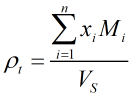Where ρt = Saturated mixture density at temperature t xi = Mole fraction of ith component Mi = Molar mass of ith component VS = Molar volume of mixture at temperature t

The molar mass values are obtained from Ref  above. The molar volume of the mixture, VS, is calculated from: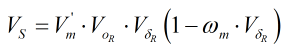Where V’m = Characteristic volume of the mixture VoR = Corresponding states function for normal fluids Vδ R = Deviation function ωm = Acentric factor of mixture

The above parameters are calculated as follows: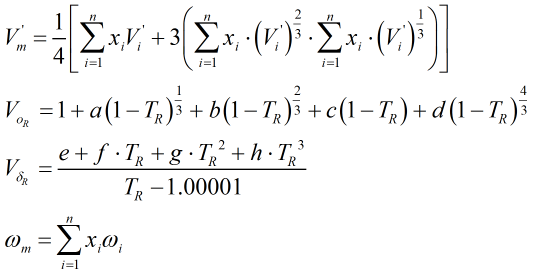Where V’i = Characteristic volume of ith component TR = Reduced temperature of the mixture ωi = Acentric factor of ith component

The constants a, b, c, d, e, f, g and h, along with the component characteristic volumes and acentric factors, are obtained from Ref  above. The reduced temperature, TR, is calculated from: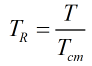Where T Absolute temperature of mixture Tcm Critical temperature of the mixture

The critical temperature of the mixture is calculated from: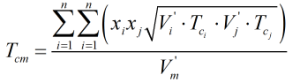Where Tci = Critical temperature of ith component Tcj = Critical temperature of jth component V’i = Characteristic volume of ith component V’j = Characteristic volume of jth component

The component critical temperatures are obtained from Ref  above.

b) “Enhanced” COSTALD The LNG mixture density is calculated using the same method as the “standard” COSTALD equation above (for LPG), with the only exception being the calculation of the critical temperature of the mixture, Tcm. For the “enhanced” COSTALD equation (for LNG only), Tcm is calculated from: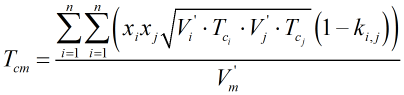where the additional interaction parameters, ki,j, are given in Ref .

c) COSTALD-Tait The compressed liquid density of light hydrocarbon mixtures is calculated from the following equation: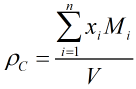where V is the compressed molar volume of the mixture given by: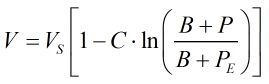Where VS = Saturated molar volume from the main COSTALD routine P = Observed pressure PE = Equilibrium vapour pressure

The constants B and C are calculated from: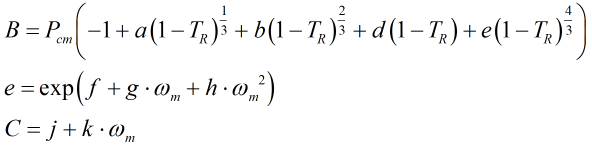where Pcm is the critical pressure of the mixture, the derivation of which is given further below. The constants a, b, d, f, g, h, j, and k are given in Ref  above.

NOTE: Ref  contains an error in stating “d” as 135.1102 (+ve), whereas the original publication from which the equations are taken give “d” as -135.1102 (-ve).

The equilibrium vapour pressure, PE, is calculated from: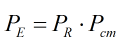where the reduced pressure, PR is given by: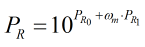and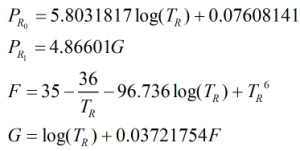and Pcm is given by:Where Zcm = Critical compressibility factor of the mixture R = Gas constant equal to 8.31441 J·mol-1·K-1 Tcm = Critical temperature of the mixture from the main COSTALD routine V’m = Characteristic volume of the mixture from the main COSTALD routine

The critical compressibility factor is given as: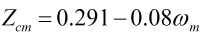Back